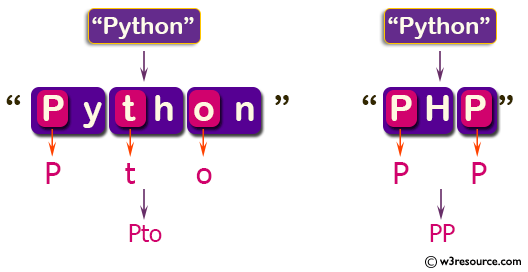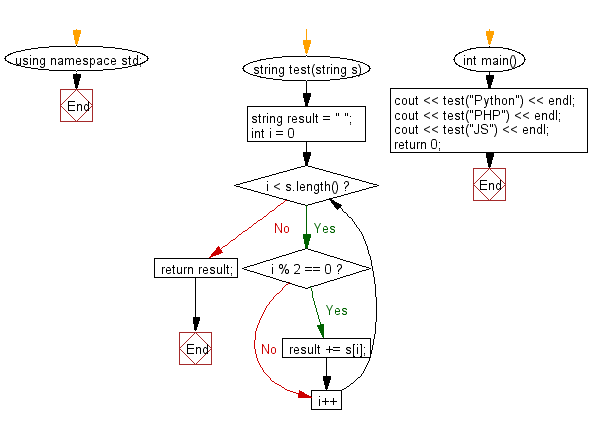﻿ C++ : New string based on every other character in a string# C++ Exercises: Create a new string made of every other character starting with the first from a given string

## C++ Basic Algorithm: Exercise-28 with Solution

Write a C++ program to create a new string made of every other character starting with the first from a given string.

Sample Solution:

C++ Code :

``````#include <iostream>
using namespace std;

string test(string s)
{
string result = " ";
for (int i = 0; i < s.length(); i++)
{
if (i % 2 == 0) result += s[i];
}
return result;
}

int main()
{
cout << test("Python") << endl;
cout << test("PHP") << endl;
cout << test("JS") << endl;
return 0;
}
``````

Sample Output:

```Pto
PP
J
```

Pictorial Presentation:Flowchart:C++ Code Editor:

Contribute your code and comments through Disqus.

What is the difficulty level of this exercise?

﻿